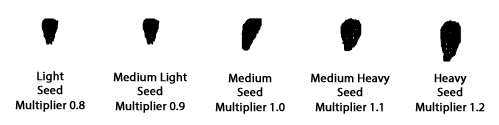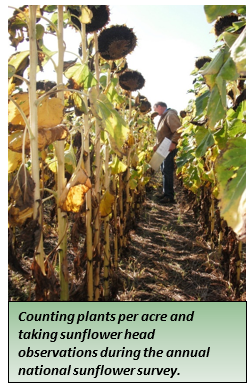Page Title

# Estimating Sunflower Yield

Body

This page was adapted from the article, "Estimating Sunflower Yield," which appeared in Crop & Pest Report on September 23, 2021.

The sunflower yield for oil sunflower, can be calculated by the number of plants per acre, the head diameter, the seed size, the number of filled seeds per head, observing how much of the center of the head is filled, and estimating the damage done by birds. The yield is calculated by multiplying 2,450 by the “multipliers” from the tables in this article.

First, estimate the plant population from a number of locations within a field, away from the headlands.

Table 1. Multiplier to be used based on sunflower plant population per acre.

 Plants per acre Multiplier 14,000 0.7 16,000 0.8 18,000 0.9 20,000 1.0 22,000 1.1 24,000 1.2

For plant populations between the numbers in Table 1, the value of the multiplier can be estimated. The next measurement is the sunflower head diameter. Measure a number of heads in the field (photo 1), and take the average. Plants with more space tend to have larger heads so it is important to take a representative field sample.Table 2.  Average sunflower head diameter in inches and multiplier to be used in formula.

 Head diameter  (inches) Multiplier Head diameter (inches) Multiplier 4.5 0.37 7.5 0.91 5.0 0.46 8.0 1.00 5.5 0.55 8.5 1.09 6.0 0.64 9.0 1.18 6.5 0.73 9.5 1.27 7.0 0.82 10.0 1.36

The next multiplier is related to the seed size. Cut a small wedge out of a few heads, thresh seed and put in small container. Take a representative seed sample from bucket and determine seed size for the multiplier (Figure 1).Figure 1. Sunflower seed size chart with multipliers.

Table 3. Seed size and multiplier to use.

 Descriptor Light (L) Medium Light (ML) Medium (M) Medium Heavy (MH) Heavy (H) Multiplier 0.8 0.9 1.0 1.1 1.2 Seed width in mm 4.2 5.2 5.8 5.8 5.8 Seed length in mm 7.3 7.8 8.9 9.9 11

Next, take 100 seeds from seed sample (in container) and determine percent filled seeds. For instance, when observing 100 seeds of which 9 seeds are either not filled or severely damaged due to insects, the multiplier will be 0.91 (= 91 % filled seeds).

The following measurement is to observe the center seed set. If there is no seed set in the center one inch of the head, multiply by 0.975; if no seeds are set in the center two inches of the head, multiply by 0.95; if there is a hole in the center of the head, multiply by 0.9. Take the average seed set from a number of plants.

Lastly observe if there is bird damage to the heads. If there is 2% damage, there is no damage for 98% of the head and the correction factor will be 0.98

Yield calculation

Yield is calculated by multiplying 2,450 by the multipliers from the tables. For example in a sunflower field with a stand of 20,000 plants per acre, average head size of 6.5 inches, a medium seed size with good seed count of 95%, and average no seed in the center of the head of one inch, and 2% bird damage you would do the following.Multipliers used: 2,450 x plant population x head size x seed size x good seed count x center seed set x no bird damage = lbs/acre.

Yield calculations for this example field would therefore be: 2,450 x 1.0 x 0.73 x 1.0 x 0.95 x 0.975 x 0.98 = 1623 lbs/acre. The yield estimate for this example is 1623 lbs per acre. The actual yield may be lower if not all heads can be harvested and/or additional bird damage will occur.

Reference:

National Sunflower Association article How to Estimate Yield

Hans Kandel